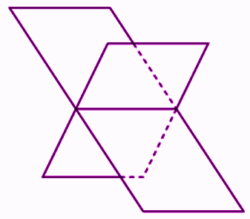# Describe line of intersection of planes

In geometry, lines as we will as planes are two basic shapes.

• A line is a set of points that stretches infinitely in opposite directions. It has only one dimension, i.e., length. The points that lie on the same line are called collinear points.
• A point is a location in a plane that has no size, i.e. no width, no length and no depth.

### Line of intersection of planes

Two planes can be related in the following ways, in a three-dimensional space.

• They can be parallel to each other
• They can be identical
• They can intersect each other

The figure given below represents two intersecting planes(1)(2)